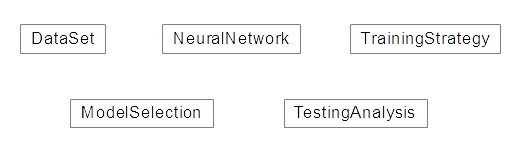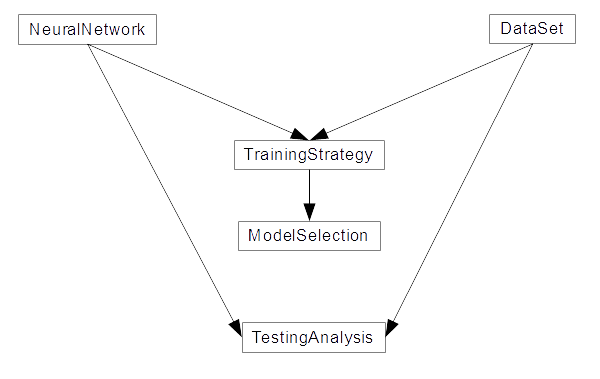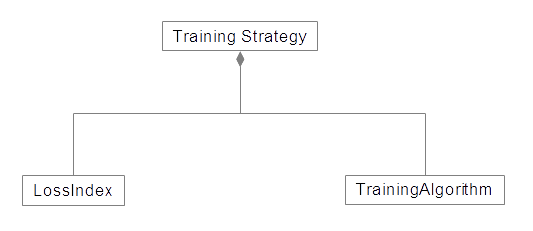#Software model of OpenNNIn this tutorial, we present the software model of OpenNN. The whole process is carried out in the Unified Modeling Language (UML). The Unified Modeling Language (UML) is a general-purpose visual modelling language used to specify, visualize, construct, and document the artefacts of a software system.

To construct a model for OpenNN, we follow a top-down development. This approach to the problem begins at the highest conceptual level and works down to the details. In this way, to create and evolve a conceptual class diagram, we iteratively model:

## 1. Classes

In colloquial terms, a concept is an idea or a thing. In object-oriented modeling, concepts are represented through classes. Therefore, a prime task is to identify the main concepts (or classes) of the problem domain. In UML class diagrams, classes are depicted as boxes. The next figure depicts a starting UML class diagram for the conceptual model of OpenNN.Conceptual diagram for OpenNN.

• DataSet: This class contains utilities dealing with processing and treatment of data.
• NeuralNetwork: This class represents the concept of a neural network; this aggregates all the different neural network concepts.
• TrainingStrategy: This class represents the concept of a training strategy for a neural network in OpenNN.
• ModelSelection: This class represents the concept of a model selection algorithm in OpenNN. It is used for finding a network architecture with maximum generalization capabilities.
• TestingAnalysis: This class represents the concept of analysis; it contains tools for testing neural networks in different learning tasks.

## 2. Associations

Once the main concepts in the model are identified, it is necessary to aggregate the associations among them. An association is a relationship between two concepts that points to some significant or interesting information.

In UML class diagrams, an association is shown as a line connecting two classes. The relevant associations among the main concepts of OpenNN are next identified to be included in the UML class diagram of the system:• TrainingStrategy - NeuralNetwork & DataSet: TrainingStrategy handles Dataset and NeuralNetwork to perform neural network training.
• ModelSelection - TrainingStrategy: ModelSelection uses TrainingStrategy to check which model gets the best results.
• TestingAnalysis - DataSet & NeuralNetwork: TestingAnalisys evaluate NeuralNetwork over a DataSet.

## 3. Compositions

Classes are usually composed of other classes. The higher-level classes manage the lower-level ones. Regarding OpenNN, the concepts of DataSet, NeuralNetwork, TrainingStrategy, ModelSelection, and TestingAnalysis, are relatively high-level structures. This means that these classes are composed of different elements.

In general, the goal of OpenNN is to encapsulate basic concepts in elementary classes and then create larger classes with broader concepts. For instance, referencing the NeuralNetwork, PerceptronLayer, ProbabilisticLayer, or other layers of neurons are subclasses enclosed in Layer. This class represents the concept of a layer from any kind of neuron. A set of Layers is necessary to build a NeuralNetwork.

### DataSet

DataSet is not composed of classes. Instead, it is a high-level class where all methods are controlled by it.

The following diagram shows the only class in DataSet:### NeuralNetwork

The next UML diagram shows the most important classes of NeuralNetwork:• PerceptronLayer: This class represents a layer of perceptrons.
• ScalingLayer: This class represents a layer of scaling neurons.
• UnscalingLayer: This class represents a layer of unscaling neurons.
• Boundinglayer: This class represents a layer of bounding neurons.
• ProbabilisticLayer: This class represents a layer of probabilistic neurons.
• LongShortTermMemoryLayer: This class represents a layer of LSTM neurons.
• RecurrentLayer: This class represents a layer of recurrent neurons.
• ConvolutionaLayer: This class represents a layer of convolutional neurons.
• PoolingLayer: This class is used to store information about the Pooling Layer in a Convolutional Neural Network (CNN).
• PrincipalComponentsLayer: This class represents the layer of principal component analysis.

### TrainingStrategy

The following diagram shows the most relevant classes in TrainingStrategy:• LossIndex: This abstract class represents the concept of the error term.
• TrainingAlgorithm: This abstract class represents the concept of a training algorithm for a neural network.

### ModelSelection

In the picture below, the elementary classes of ModelSelection are shown:• InputSelection: This abstract class represents the concept of an input selection algorithm for a neural network.
• NeuronsSelection: This abstract class represents the concept of neurons selection algorithm for a neural network.

## 4. Derived classes

In object-oriented programming, some classes are designed only as a parent from which subclasses may be derived, but which is not itself suitable for instantiation. This is said to be an abstract class, as opposed to a concrete class, which is suitable for instantiation.

The derived class contains all the base class features but may have new features added or redefine existing features. Associations between a base class and a derived class are of the kind is a.

### TrainingStrategy

The classes: Mean Square error, CrossEntropyError, MinkowskiError, NormalizedSquaredError, SumSquaredError, WeightedSquareError are derived from LossIndex; all of them have in common that they inherit the characteristics of the base class. However, each of these classes also introduces new features, such as its definition of error.Likewise, GradientDescent, AdaptativeMomentEstimation, ConjugateGradient, QuasiNewtonMethod, LevenbergMarquadtAlgorithm, and StochasticGradientDescent are a set of concrete classes that have inherited all the characteristics of the abstract class TrainingAlgotihm.### ModelSelection

Like TrainingStrategy, ModelSelection is composed of 2 abstract classes InputSelectionAlgorithm and OrderSelectionAlgorithm. Both have their own derived classes that inherit the features of each one.

InputSelectionAlgorithm is an abstract class composed of 3 concretes classes: GrowingInputs, GeneticAlgorithm, PruningInputs.NeuronsSelection is an abstract class composed of a concrete class: IncrementalNeurons.## 5. Members and methods

A member (or attribute) is a named value or relationship that exists for all or some class instances. A method (or operation) is a procedure associated with a class.

In UML class diagrams, classes are depicted as boxes with two sections: the top lists the class's attributes and the bottom lists the operations. The prominent members and methods of the different OpenNN classes are described throughout this manual.

The most important attributes and methods of the DataSet class:The essential attributes and methods of NeruralNetwork class are:In the following chart, the most important attributes of the training strategy class are shown:The most important attributes and methods of the ModelSelection class are:The next box shows the essential attributes and methods of TestingAnalisys class:## Bibliography

• C. Bishop. Neural Networks for Pattern Recognition. Oxford University Press, 1995.
• H. Demuth, M. Beale, and M. Hagan. Neural Network Toolbox User's Gide. The MathWorks, Inc., 2009.
• S. Haykin. Neural Networks: A Comprehensive Foundation. Prentice Hall.
• R. Lopez. Neural Networks for Variational Problems in Engineering. Ph.D. Thesis, Technical University of Catalonia, 2008.